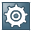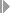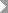Show TOC

###Definition of Dimensions and Units of MeasurementYou use dimensions to group units of measurement. You need units of measurement to display product quantities, for example, for freight unit building rule (FUB rules), and to describe the capacity of vehicle resources (see Freight Unit Building Rule and Vehicle Resource).Note

The system might display quantities with 14 decimal digits. If you want the system to round quantities to a specific number of decimal digits, follow the instructions described in SAP Note 1132219.

End of the note.

#### Prerequisites

• You have checked in Customizing for SAP NetWeaver underSAP NetWeaverGeneral SettingsCheck Units of Measurementthat the dimensions delivered as a standard and the associated units of measurement are complete.

• When using FUB rules, the system checks if the forwarding order contains the relevant quantities based on the FUB rules. If the forwarding order does not contain the relevant quantities, the system uses the product data. For this purpose, you have defined the following in the product master data:

• Base unit of measure (BUoM)

• Conversion factors

You need conversion factors to convert the base units of measure into the required units of measurement. You define these in the product master on the Units of Meas. tab page. For more information, see Definition of Products.

If you have not defined a conversion factor, the system cannot take split quantities or rounding values into account when building freight units from business documents (forwarding orders, for example). In this case, it sets the quantities to zero.

#### Example

##### Examples for Dimensions and Units of Measurement

The following table provides you with examples of dimensions and of some units of measurement you can assign to them.

 Dimension Units of Measurement Mass Kilogram; milligram Volume Cubic centimeter; cubic decimeter
##### Example for the Conversion of Units of Measurement

The following units of measurement are predefined as a default:

• Kilogram (dimension mass)

• Cubic decimeter (dimension volume)

You also define the following unit of measurement:

• Pallet (no dimension)

Your forwarding order contains product A and product B each of which have the quantity "1 piece". You have defined piece as BUoM for both products.

You have defined the following conversion factors for product A:

• 1 piece = 2 kg

• 1 piece = 3 dm³

You have defined the following conversion factor for product B:

• 1 piece = 0.5 pal

In this case, product A is displayed with quantity 2 kg or 3 dm³. In the unit of measurement pallet (pal), the quantity 0 is displayed because you have not defined a conversion factor.

Product B is displayed with the quantity 0.5 pal. Since you have not defined conversion factors for kilogram or cubic decimeter, no conversion occurs. The system displays the value 0.